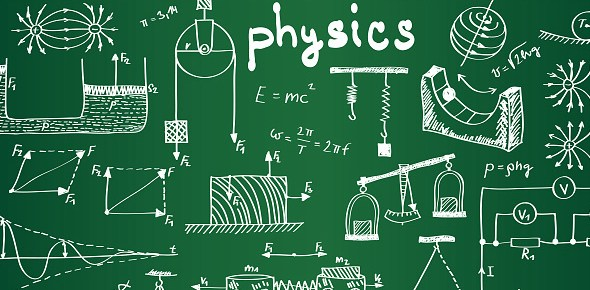# Physical Science Chapter 18

21 Questions | Total Attempts: 98SettingsPhysical Science. Yes.

• 1.
Increasing temperature increases disorder and entropy.
• A.

True

• B.

False

• 2.
Irreversible processes are always spontaneous and occur on their own without any intervention.
• A.

True

• B.

False

• 3.
From our current understanding, sometime in the future engineers will design a car that is completely efficient (a car that creates no additional disorder in the universe).
• A.

True

• B.

False

• 4.
Gravitational potential energy is a more ordered form of energy than electrical potential energy.
• A.

True

• B.

False

• 5.
A salt shaker and a pepper shaker get knocked off the table and break on the floor. Separating the salt and pepper can be considered an irreversible process.
• A.

True

• B.

False

• 6.
An ice cube melts in a warm glass of water. This is an example of an ______ process.
• 7.
Complete conversion of one energy form to another energy form takes place only if the energy converts to a __________ energy form.
• 8.
The universe is the combination of a(n) _______ plus its _________.
• 9.
Everything outside what we have defined to be the system.
• 10.
A process that goes in only one direction; its effects cannot be undone. Most processes that occur in nature are this way.
• 11.
A process that goes both forward and backward at the same time.
• 12.
A small piece of the world around which we mentally draw a box and upon which we focus our attention.
• 13.
A quantitative measure of disorder. It increases as the disorder increases.
• 14.
Disorder in the universe increases or stays the same any time a process occurs.
• 15.
Overall disorder remains unchanged in
• A.

Reversible processes

• B.

Chemical processes

• C.

Mechanical processes

• D.

Irreversible processes

• 16.
If analyzing a fridge in relationship to its surroundings, what would the fridge be considered?
• A.

Entropy

• B.

Surroundings

• C.

System

• D.

Both b and c

• 17.
Which of the following is a reversible process?
• A.

A man diving into a pool

• B.

Water falling down Niagara Falls

• C.

A pendulum swinging in a frictionless environment

• D.

Scrambling eggs

• E.

None of the above

• 18.
Which of the following processes is closest to an ideal reversible process?
• A.

A golf ball falls into med and sticks there

• B.

Water evaporates from a glass sitting on the kitchen table

• C.

A book sliding on a horizontal table comes to rest because of friction

• D.

A ripe peach rots in the Sun

• E.

An ice cube changes shape while floating in a glass of water at 0 degrees C

• 19.
An auto engine cannot convert all of its fuels chemical potential energy into macroscopic kinetic energy because
• A.

Energy would not be conserved

• B.

Energy would be conserved

• C.

Mass would not be conserved

• D.

Disorder would be decreased

• 20.
The Law of Increasing Disorder places limits on the efficiency of energy conversion. Which of the following is allowed?
• A.

100% conversion of ambient temperature thermal energy to kinetic energy

• B.

100% conversion of chemical potential energy to kinetic energy

• C.

100% conversion of sunlight to kinetic energy

• D.

100% conversion of electricity to ambient temperature thermal energy

• E.

100% conversion of ambient temperature thermal energy to chemical potential energy

• 21.
Of the quantities listed, the one associated with the most disorder is
• A.

Chemical potential energy

• B.

Gravitational potential energy

• C.

Macroscopic kinetic energy

• D.

Nuclear potential energy

• E.

Thermal energy in a glass of water

Related TopicsBack to top